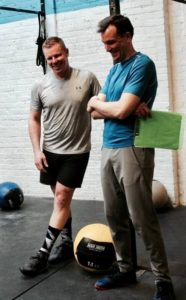## Thursday, June 1, 2017

### Thursday, June 1, 2017as many rounds as possible in 20 minutes:
400m run
20x burpee

### 1 Comment

1.–Scores–
Borja G.: 5 rounds + 7x burpee
Kate H.: 3 rounds + 12x burpee
Blaine P.: 5 rounds + 350m
Matthew W.: 6 rounds + 100m
Michael F.: 6 rounds + 100m
Kevin K.: 4 rounds + 7x burpee
Ralph A.: 3 rounds + 7x burpee (sub: 3/4x burpee)
Hari P.: 5 rounds + 200m
John L.: 6 rounds + 13x pushup (sub: 200m row/1′ plank, pushup)
Brian M.: 5 rounds + 350m
Anna G.: 4 rounds + 200m
Stephen C.: 5 rounds + 250m
Carla G.: 5 rounds + 100m
Jason S.: 5 rounds
Thomas R.: 2 rounds + 7x burpee (sub: 1/2x burpee)
Walt C.: 4 rounds + 6x burpee
Diana G.: 4 rounds (sub: 3/4x burpee)
Seth C.: 4 rounds + 100m
Peter Mc.: 5 rounds + 400m
Jerry I.: 4 rounds + 1x burpee
Luke B.: 5 rounds + 375m
David G.: 4 rounds + 300m
Chase H.: 5 rounds + 16x burpee
Elise C.: 5 rounds + 100m
Tara S.: 5 rounds + 100m
Carl S.: 5 rounds + 100m
Ralph B.: 4 rounds + 14x burpee
Allie R.: 4 rounds + 100m
Shawn J.: 4 rounds + 3x burpee
Jo C.: 7 rounds + 10″ (sub: 2′ bike, sit-up/glute bridge)
Buffy M.: 4 rounds + 75m (sub: 3/4x burpee)
Kevin M.: 4 rounds + 50m (sub: 3/4x burpee)
Dave R.: 5 rounds + 400m
Dave K.: 5 rounds + 5x burpee
Udo D.: 4 rounds + 350m
Saumil S.: 4 rounds + 6x burpee
Liz L.: 5 rounds
Josh B.: 5 rounds + 150m
James H.: 7 rounds + 60″ (sub: 2′ bike, 10x hanging knee raise/10x glute bridge (even), pushup (odd))
Danielle Z.: 3 rounds + 350m (sub: 1/2x burpee)
Francisco L.: 4 rounds + 7x burpee
Sebastian E.: 5 rounds + 50m
Josh M.: 5 rounds + 275m
Candida M.: 5 rounds + 100m
Sean Mc.: 4 rounds + 10x burpee
Travers O.: 5 rounds + 5x burpee
Rob M.: 5 rounds + 1x burpee (sub: 3/4x burpee)
Edgar O.: 4 rounds + 375m (sub: 3/4x burpee)
Amelia F.: 4 rounds + 13x burpee
Jesse M.: 3 rounds + 10x burpee
Stephen K.: 4 rounds + 350m (sub: 3/4x burpee)
Jim D.: 3 rounds + 5x burpee (sub: 3/4x burpee)
Rachel B.: 4 rounds + 350m
Jin D.: 5 rounds
Jarod F.: 4 rounds (sub: 1/2x burpee)
Kat S.: 4 rounds + 350m (sub: 3/4x burpee)
Chris T.: 5 rounds + 100m
John P.: 6 rounds + 150m
Brenda Y.: 4 rounds + 12x burpee (sub: 3/4x burpee)
Mark Co.: 4 rounds + 4x burpee
Patrick J.: 4 rounds + 400m
Miranda B.: 5 rounds + 40″ (sub: 2′ bike, 3/4x burpee)